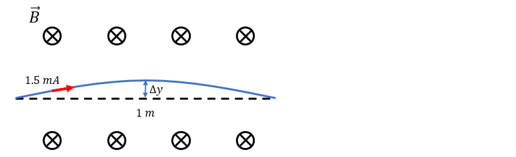# Problem: A 1m, straight wire carrying a 1.5 mA current in the presence of a perpendicular, 2mT magnetic field will feel a force due to that magnetic field. Because of this force, the wire should deform, as shown in the following figure. However, this deformation causes stretching in the wire (obviously the arc is a further distance than 1m), and if the wire is elastic, then there will be some elastic force that resists stretching. If we can treat the wire like we treat a spring, and say the elastic force is proportional to the vertical displacement, Fel = -kΔy, how much could the wire stretch if k = 300 N/m? Assume that the wire is still essentially 1m long after stretching.

###### FREE Expert Solution
90% (333 ratings)
###### Problem Details

A 1m, straight wire carrying a 1.5 mA current in the presence of a perpendicular, 2mT magnetic field will feel a force due to that magnetic field. Because of this force, the wire should deform, as shown in the following figure. However, this deformation causes stretching in the wire (obviously the arc is a further distance than 1m), and if the wire is elastic, then there will be some elastic force that resists stretching. If we can treat the wire like we treat a spring, and say the elastic force is proportional to the vertical displacement, Fel = -kΔy, how much could the wire stretch if k = 300 N/m? Assume that the wire is still essentially 1m long after stretching.